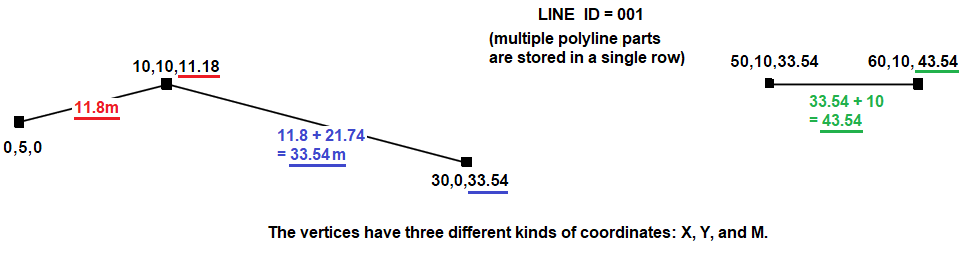# SQL & PL/SQL

## Code Review: Update polyline vertices with cumulative length of lineI have a multi-part polyline in a row in an Oracle 18c table. The polyline's geometry information is stored in a SHAPE column; the datatype is a user-defined spatial type called SDE.ST_Geometry.
I've written a PL/SQL function call `m_as_length()` that replaces a coordinate in the polyline's vertices with the cumulative length of the line. The coordinate is called an "M" coordinate (aka a "Measure-value"). M coordinates are similar to X and Y coordinates, but are used for specialized linear referencing purposes.

``````Input:
MULTILINESTRING M (( 0.0 5.0 -100000.0, 10.0 10.0 -100000.0, 30.0 0.0 -100000.0),( 50.0 10.0 -100000.0, 60.0 10.0 -100000.0))
--select sde.st_astext(shape) from polylines

Output:
MULTILINESTRING M ((0 5 0, 10 10 11.18, 30 0 33.54),(50 10 33.54, 60 10 43.54))
--select m_as_length(shape) from polylines
``````

Question:
I'm a novice when it comes to PL/SQL. Can the `m_as_length()` function be improved?

``````with
function pythagoras(x1 in number, y1 in number, x2 in number, y2 in number) return number is
begin
return round(  sqrt(power(x2 - x1, 2) + power(y2 - y1, 2))  ,2);
end;

function m_as_length(shape in sde.st_geometry) return varchar2
is
result              varchar2(32767);
vertex              varchar2(32767);
vertex_set          varchar2(32767);
i                   number;
j                   number;
num_parts           number;
num_points          number;
oldX                number;
oldY                number;
newX                number;
newY                number;
line_len            number;
begin
num_parts           := sde.st_geometry_operators.st_numgeometries_f(shape);
oldX                := sde.st_geometry_operators.st_x_f(sde.st_geometry_operators.st_startpoint_f(sde.st_geometry_operators.st_geometryN_f(shape,1)));
oldY                := sde.st_geometry_operators.st_y_f(sde.st_geometry_operators.st_startpoint_f(sde.st_geometry_operators.st_geometryN_f(shape,1)));
line_len            := 0;

for i in 1..num_parts
loop
num_points      := sde.st_geometry_operators.st_numpoints_f(sde.st_geometry_operators.ST_GeometryN_f(shape,i));
vertex_set      := null;
for j in 1..num_points
loop
newX        := sde.st_geometry_operators.st_x_f(sde.st_geometry_operators.st_pointn_f(sde.st_geometry_operators.st_geometryn_f(shape,i),j));
newY        := sde.st_geometry_operators.st_y_f(sde.st_geometry_operators.st_pointn_f(sde.st_geometry_operators.st_geometryn_f(shape,i),j));
if j <> 1 then
line_len := line_len + pythagoras(oldX, oldY, newX, newY);
end if;
oldX        := newX;
oldY        := newY;
vertex      := newX || ' ' || newY || ' ' || line_len;
vertex_set  := vertex_set || vertex || ', ';
end loop;
vertex_set      := '(' || rtrim((vertex_set),', ') || '),';
result          := result || vertex_set;
end loop;
result              := 'MULTILINESTRING M (' || rtrim((result),',') || ')';
return result;
end;
select
m_as_length(shape)
from
polylines
``````

Related:
Use SDE.ST_GEOMETRY functions in a custom function
Explains why the function calls are so verbose: `sde.st_geometry_operators.st_numgeometries_f`.
`st_numgeometries`
`st_x`
`st_startpoint`
`st_geometryN`
`st_numpoints`
`st_pointn`